Next: 6.2.2 Poisson-Boltzmann Model with Up: 6.2 Modeling BioFETs Previous: 6.2 Modeling BioFETs

## 6.2.1 Poisson-Boltzmann Model

The often employed Poisson-Boltzmann model is a good approximation for electrolytes from several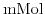upwards. Assuming that the dissolved buffer ions are in thermodynamical equilibrium with their environment and their concentration only depends on the local potential, the charge contribution from the buffer can be written as sum over all ionic species of the buffer weighted with Boltzmann type terms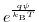and their valences. The Poisson-Boltzmann model has been already introduced in Section 5.3 and is now generalized to a two-dimensional formulation6.1: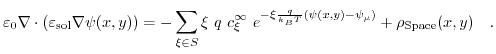(6.1)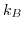denotes Boltzmann's constant,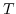the temperature in Kelvin, and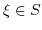, where S contains the valences of the ions in the electrolyte.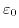is the permittivity of vacuum, and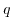denotes the elementary charge.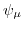is the chemical potential.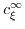is the ion concentration in equilibrium, while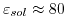is the relative permittivity of water.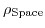represents the average space charge density in the simulation zone, where the charged macromolecules are held.

#### Footnotes

... formulation6.1
In general this formulation can be extended to three dimensions, but due to the device geometry two-dimensional cuts are sufficient.Next: 6.2.2 Poisson-Boltzmann Model with Up: 6.2 Modeling BioFETs Previous: 6.2 Modeling BioFETs

T. Windbacher: Engineering Gate Stacks for Field-Effect Transistors